# Scripts/functions to split/cut model with an axis-orthogonal planeClassicListThreaded4 messagesOpen this post in threaded view
|

## Scripts/functions to split/cut model with an axis-orthogonal plane

 This post was updated on . ************* UPDATE ************* The functionality provided by the scripts described in this post has been implemented and improved in FEATool version 1.12.4 build 20.09.249 as "Split Object" option in the "Geometry" menu.-Randal ********************************** This post http://forum.featool.com/Slice-plane-tool-in-Geometry-Mode-tp508.html mentions functionality in the geometry mode that would split or cut a model along a planar surface. When a problem's geometry model contains more than one or two objects/subdomains, I find that doing it manually, according to the method outlined in the post, although effective in accomplishing a slice, to be tedious and error prone task: for each object encountered by the cut plane, duplicate it in place then create 2 blocks which share a common face in the slice plane and together enclose the entire model, and finally subtract one block from the object and the other block from its duplicate I have implemented a set of 3 scripts that I believe does this for me, i.e., takes the geometry model from the current FEATool session problem (fea.geom) and produces a geometry model in the MATLAB workspace that has been sliced/cut by a designated axis-perpendicular/orthogonal plane. It then requires only a manual import into the FEATool Geometry mode. Here are the 3 files that support this functionality, followed by their help text: geom_slice_model_gui.m>> help geom_slice_model_gui geom_slice_model_gui() - a wrapper function for geom_slice_model Exports fea from current FEATool session, prompts for an axis-orthogonal slice plane selection ('XY', 'XZ', or 'YZ'), an invariant axis coordinate variable value, and other argument(s) from a popup window. After checking the validity of the user input, it calls "geom_slice_model" which slices the fea.geom geometry along the specified plane. If a normal status is returned, it copies the returned GEO to the MATLAB base workspace with the name fea_geom_sliced_SUFFIX, wher SUFFIX indicates the slice plane and invariant axis coordinate, val.(e.g.,'YZ' plane at x=1.2 --> YZ_1_2) orthoplane: 'XY', 'YZ', or 'XZ' to denote orientation of the slice plane val: The value of the invariant coordinate in the plane (e.g., for 'XY', z is invariant) e.g., 0.5 do_plot: If true, the resulting geometry model is plotted before and after slice processing. See also: geom_slice_model geom_bounds_and_size geom_slice_model.m>> help geom_slice_model [GOBJ_SLICED, stat] = geom_slice_model ( GOBJ, orthoplane, val, plot ) slices entire model GOBJ with orthogonal (axis-perpendicular) plane Slices an FEATool GOBJ with a [cut-]plane effectively dividing any object that it interects at the plane of intersection, thus creating two distinct components which share an internal boundariy at the intersection. These boundaries my be integrated over in the Postprocessing mode. The component pieces generated by the slice may be subsequently deleted, transformed, or combined with other component objects to further develop the model geometry in the usual manner. The first argument is a geometry object such as fea.geom, orthoplane and val define the cutting plane, and plot enables the plotting of figures including the unmodified model that is input (GEOB) and the output sliced/cut model geometry (GEOB_SLICED). The final plot adds a highlighed and enlarged component to illustrate the position of the underlying slice/cut. Inputs: GOBJ : a geometric object struct as in the fea struct member geom(fea.geom). orthoplane : 'XY', 'YZ', or 'XZ' to denote orientation of the slice plane val : The value of the invariant coordinate in the plane (e.g., for 'XY', z is invariant) e.g., 0.5 plot : [true] If omitted or true, the geometry model is plotted at various points, including before and after the slice is done. Example : geom_slice_model(fea.geom, 'XY', 0.5, true) geom_slice_model(mygeom, 'XY', 0.5) Outputs: GOBJ_SLICED : the resulting sliced geometry model. stat : 0 : normal return. GOBJ_SLICED output variable is set to result. -1 : wrong number of arguments. -2 : invalid slice plane designation. (orthoplane) -3 : invalid slice plane location. val places it outside the model GOBJ Note: Each time a plot is displayed, there is a delay of about 2 seconds in the processing to allow for time to view the plot. This is governed by a variable, dwell, that is passed as an argument to the "pause" function. It is set near the top of the code and can be changed to a more suitable value (including 0). See also geom_slice_model_gui geom_bounds_and_size geom_bounds_and_size.m>> help geom_bounds_and_size [P_MIN, P_MAX, SIZ] = geom_bounds_and_size (GOBJ) Calculates the bounds and extent of input geomtry object, GOBJ, and returns: 1) Points P_MIN & P_MAX, the coordinates of diagonally oposite vertexes of a rectangular block which wholly contains all objects in GOBJ and 2) SIZ, the x, y, and z, lengths of the box bounded by P_MIN & P_MAX. The workflow is, from the MATLAB command window, execute the wrapper function: >> geom_split_model_gui Then you should see a prompt for the parameters: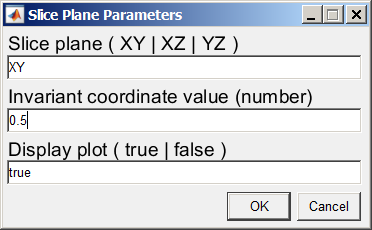Once the parameters are accepted (assuming the Display plot option is 'true') you should see a figure with the input geometry that is to be sliced (taken from the current FEATool session):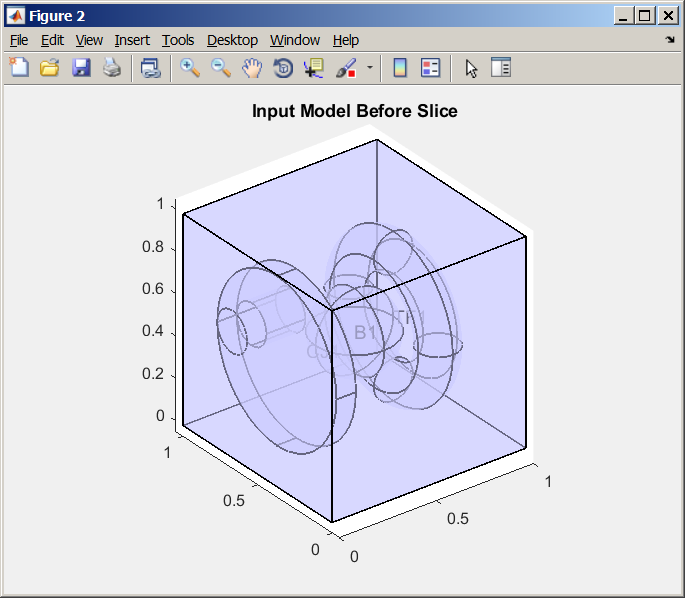Then after the passing of the "dwell" time (see help for geom_slice_model), and the slice has been done, the plot changes to that of the result that is output to the MATLAB workspace showing the cut plane: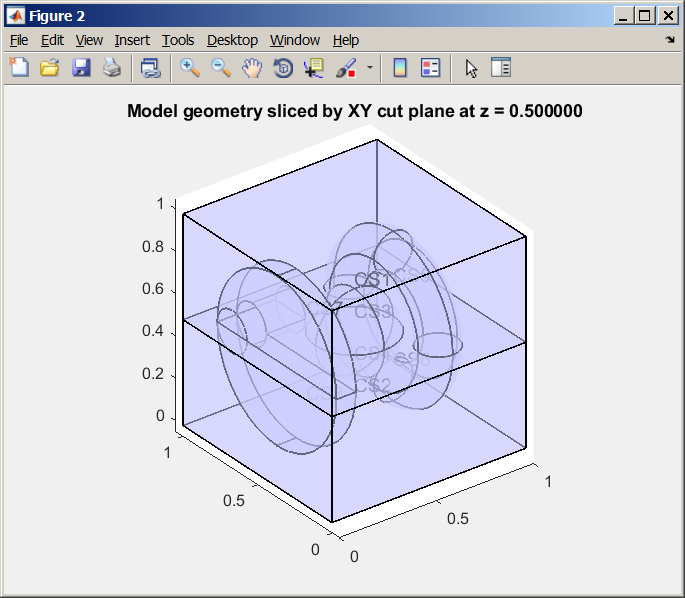After the "dwell" time another plot is shown.  It is the same as the result plot just shown, with an added illustration of where the slice plane is located.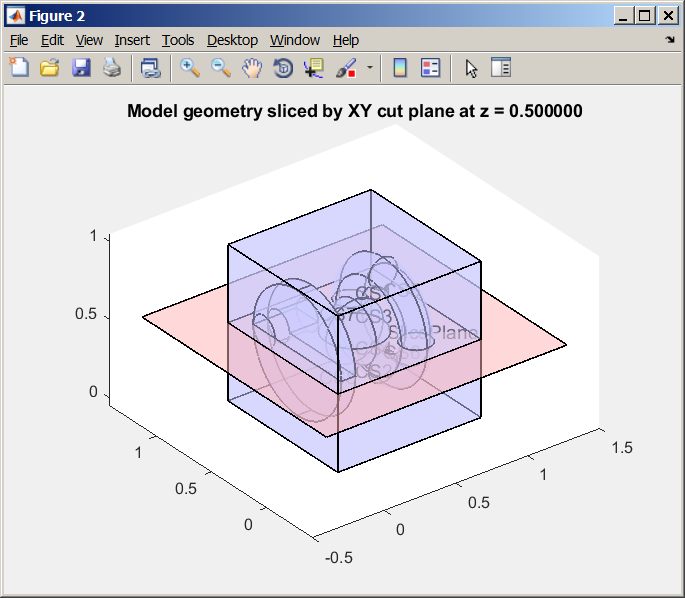Also displayed when the slice is complete is a summary message: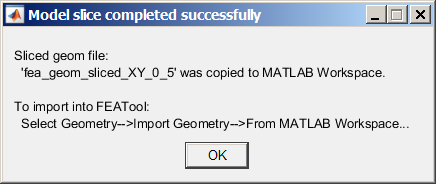An example of what is output to the MATLAB command window is: >> geom_slice_model_gui Info: geom_slice_model_gui:, fea exported from current FEATool session, fea.geom used as Model to be sliced. Slice/Cut plane input parameters: Slice Plane : XY Slice Loc'n : 0.5 Display Plot: true Sliced geom was copied to Workspace: 'fea_geom_sliced_XY_0_5' To import into FEATool: Select Geometry-->Import Geometry-->From MATLAB Workspace... To continue with the sliced model in FEATool, import the sliced geometry Select from the FEATool menu: Geometry-->Import Geometry-->From MATLAB Workspace... ... and locate the exported file. If a problem is detected with an input parameter, the following should be displayed: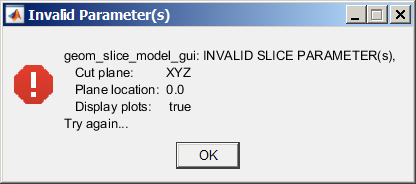If the slice plane was defined so that it does not intersect any of the model volume at all, then an error window is displayed: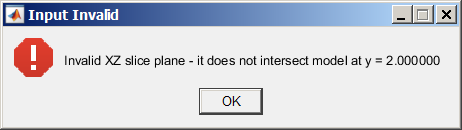...and a plot illustrates the invalid request: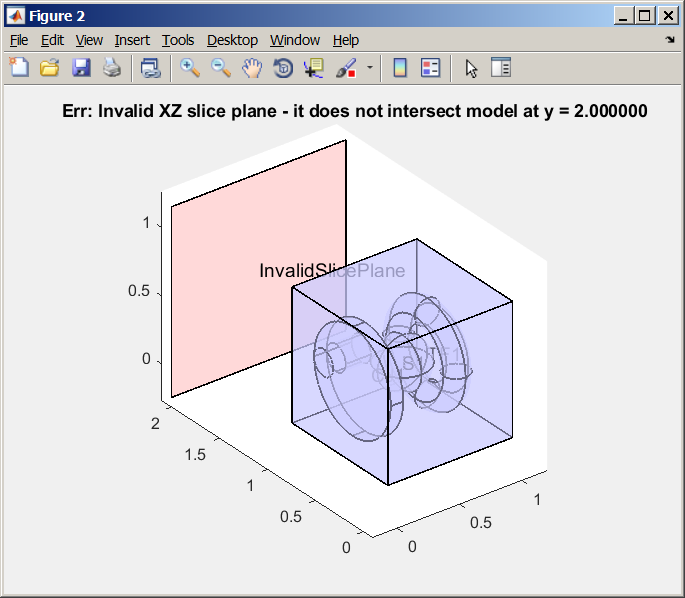Anyone who would like to try it, please do.  I'd appreciate any feedback, comments, questions, corrections, or suggestions. Kind regards, Randal
Open this post in threaded view
|

## Re: Scripts/functions to split/cut model with an axis-orthogonal plane

 Administrator Thank you for sharing your work Randall. Very impressive even with a GUI interface. Eventually I plan to incorporate slice and other operations in the geometry engine which should be more efficient and faster, but I will certainly have a look and try your version out.